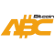Bitcoin ABC  0.22.12 P2P Digital Currency
assumptions.h
Go to the documentation of this file.
1 // Copyright (c) 2009-2010 Satoshi Nakamoto
2 // Copyright (c) 2009-2019 The Bitcoin Core developers
5
6 // Compile-time verification of assumptions we make.
7
8 #ifndef BITCOIN_COMPAT_ASSUMPTIONS_H
9 #define BITCOIN_COMPAT_ASSUMPTIONS_H
10
11 #include <climits>
12 #include <cstdint>
13 #include <limits>
14 #include <type_traits>
15
16 // Assumption: We assume that the macro NDEBUG is not defined.
17 // Example(s): We use assert(...) extensively with the assumption of it never
18 // being a noop at runtime.
19 #if defined(NDEBUG)
20 #error "Bitcoin cannot be compiled without assertions."
21 #endif
22
23 // Assumption: We assume a C++17 (ISO/IEC 14882:2017) compiler (minimum
24 // requirement).
25 // Example(s): We use std::make_unique() through the codebase.
26 // Note: MSVC does not report the expected __cplusplus value due to
27 // legacy reasons.
28 #if !defined(_MSC_VER)
29 // N3936* §16.8 [cpp.predefined]/p1::
30 // "The name __cplusplus is defined to the value 201402L when compiling a C++
31 // translation unit."
32 static_assert(__cplusplus >= 201703L, "C++17 standard assumed");
33 #endif
34
35 // Assumption: We assume the floating-point types to fulfill the requirements of
36 // IEC 559 (IEEE 754) standard.
37 // Example(s): Floating-point division by zero in ConnectBlock,
38 // CreateTransaction
39 // and EstimateMedianVal.
40 static_assert(std::numeric_limits<float>::is_iec559, "IEEE 754 float assumed");
41 static_assert(std::numeric_limits<double>::is_iec559,
42  "IEEE 754 double assumed");
43
44 // Assumption: We assume floating-point widths.
45 // Example(s): Type punning in serialization code
46 // (ser_{float,double}_to_uint{32,64}).
47 static_assert(sizeof(float) == 4, "32-bit float assumed");
48 static_assert(sizeof(double) == 8, "64-bit double assumed");
49
50 // Assumption: We assume integer widths.
51 // Example(s): GetSizeOfCompactSize and WriteCompactSize in the serialization
52 // code.
53 static_assert(sizeof(short) == 2, "16-bit short assumed");
54 static_assert(sizeof(int) == 4, "32-bit int assumed");
55
56 // Assumption: We assume 8-bit bytes, because 32-bit int and 16-bit short are
57 // assumed.
58 static_assert(CHAR_BIT == 8, "8-bit bytes assumed");
59
60 // Assumption: We assume uint8_t is an alias of unsigned char.
61 // char, unsigned char, and std::byte (C++17) are the only "byte types"
62 // according to the C++ Standard. "byte type" means a type that can be used to
63 // observe an object's value representation. We use uint8_t everywhere to see
64 // bytes, so we have to ensure that uint8_t is an alias to a "byte type".
65 // http://eel.is/c++draft/basic.types
66 // http://eel.is/c++draft/basic.memobj#def:byte
67 // http://eel.is/c++draft/expr.sizeof#1
68 // http://eel.is/c++draft/cstdint#syn
69 static_assert(std::is_same<uint8_t, unsigned char>::value,
70  "uint8_t is an alias of unsigned char");
71
72 // Assumption: We assume size_t to be 32-bit or 64-bit.
73 // Example(s): size_t assumed to be at least 32-bit in
74 // ecdsa_signature_parse_der_lax(...).
75 // size_t assumed to be 32-bit or 64-bit in MallocUsage(...).
76 static_assert(sizeof(size_t) == 4 || sizeof(size_t) == 8,
77  "size_t assumed to be 32-bit or 64-bit");
78 static_assert(sizeof(size_t) == sizeof(void *),
79  "Sizes of size_t and void* assumed to be equal");
80
81 // Some important things we are NOT assuming (non-exhaustive list):
82 // * We are NOT assuming a specific value for std::endian::native.
83 // * We are NOT assuming a specific value for std::locale("").name().
84 // * We are NOT assuming a specific value for
85 // std::numeric_limits<char>::is_signed.
86
96 static_assert((int64_t(-1) >> 1) == int64_t(-1),
97  "Arithmetic right shift assumed");
98
103 static_assert((int64_t(-10) & 0xffff) == 0xfff6, "2-complement assumed");
104
105 #endif // BITCOIN_COMPAT_ASSUMPTIONS_H